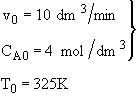# Chapter 8 Self Test

## Second Order Reaction in a CSTR.

The acid catalyzed irreversible liquid phase reaction

A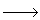B

is carried out adiabatically in a CSTR. The reaction is second order in A. The feed, which is equal molar in water (which contains the catalyst) and A, enters the reactor at a temperature of 52˚C and a total volumetric flow rate of 10 dm3/min. The concentration of A entering the reactor is 4 molar.

a) What is the reactor volume to achieve 80% conversion

b) What conversion can be achieved in a 1000 dm3 CSTR? What is the exit temperature?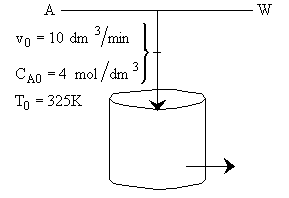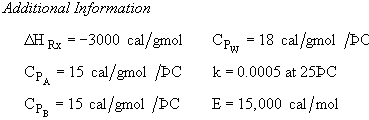Part (a)

Hint 1: What is the combined mole balance, rate law and stoichiometry that gives the reactor value as a function of temperature and conversion?

Hint 2: What is the equation that gives X, solely as a function of t, k, and CA0?

Hint 3: What is the equation that one obtains from the energy balance that gives X as a function of T?

Hint 4: What is the specific reaction rate 3?

Hint 5: What CSTR reactor volume is necessary to achieve 80% conversion?

Part (b)

Hint 1: Sketch the conversion obtained from the mole balance and energy balance as a function of T.

Hint 2: Write a Polymath program to obtain the exit conversion and temperature. Use the output to plot XEB and EMB.

#### Hint 1

Mole Balance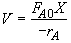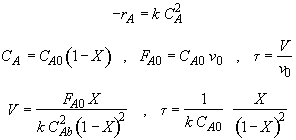#### Hint 2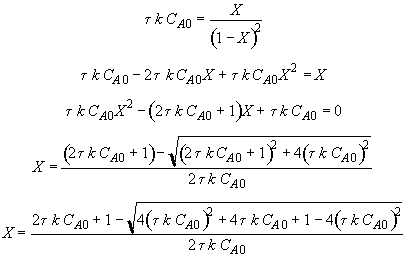Let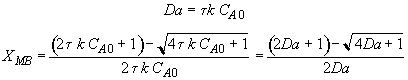#### Hint 3

Energy Balance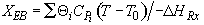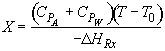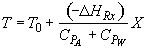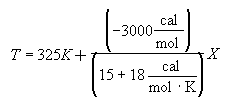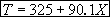For 80% conversion T = 325 + 72.7 = 397.7

#### Hint 4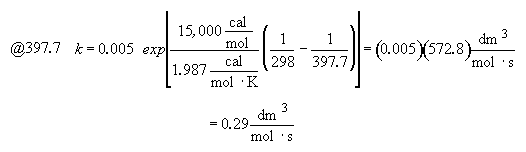#### Hint 5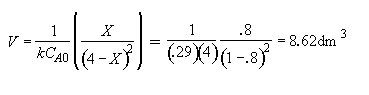Part (b)  What conversion can be achieved in a 1000 dm3 CSTR?

#### Hint 1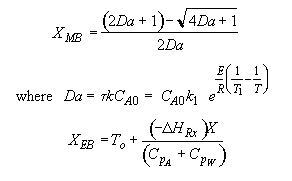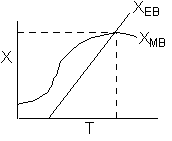The exit temperature and conversion are determined from the intersection of XEB and XMB

#### Hint 2

We can generate XEB and X vs. T curves by incrementing T and calculating the conversion.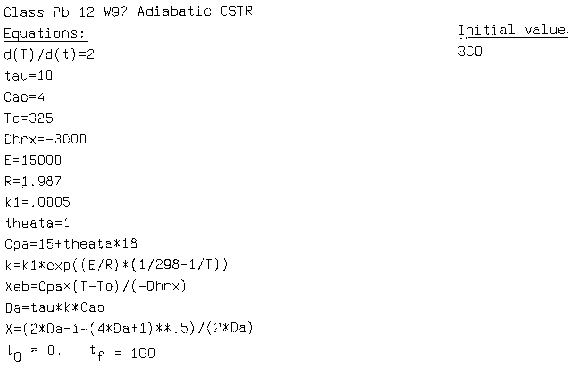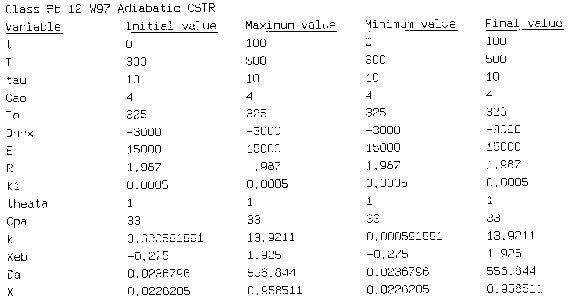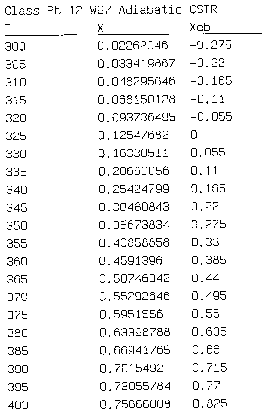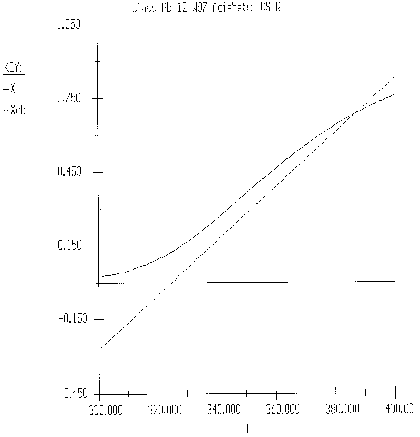Equation for Figure